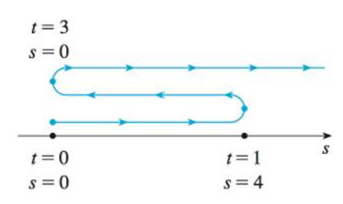Chapter 3.7, Problem 4E

Chapter
Section
Textbook Problem

A particle moves according to a law of motion s = f(t), t ≥ 0, where t is measured in seconds and s in feet.(a) Find the velocity at time t.(b) What is the velocity after 1 second?(c) When is the particle at rest?(d) When is the particle moving in the positive direction?(e) Find the total distance traveled during the first 6 seconds.(f) Draw a diagram like Figure 2 to illustrate the motion of the particle.(g) Find the acceleration at time t and after 1 second.(h) Graph the position, velocity, and acceleration functions for 0 ≤ t ≤ 6.(i) When is the particle speeding up? When is it slowing down?FIGURE 2f(t) = t2e–t

(a)

To determine

To find: The velocity at time t.

Explanation

Given:

The given equation is as below.

s=f(t)=t2et (1)

Calculation:

Calculate the velocity at time t.

Differentiate the equation (1) with respect to time.

v(t)=f'(t)=t2(et)+et(2t)=

(b)

To determine

To find: The velocity after 1 second.

(c)

To determine

To find: The time when particle at rest.

(d)

To determine

To find: The particle moving in the positive direction.

(e)

To determine

To find: The total distance traveled during the first 6 seconds.

(f)

To determine

To find: The diagram to illustrate the motion of the particle.

(g)

To determine

To find: The acceleration at time t and after 1 second.

(h)

To determine

To sketch: The graph the position, velocity, and acceleration function for 0t6.

(i)

To determine

To find: The time when the particle is speeding up and slowing down.

Still sussing out bartleby?

Check out a sample textbook solution.

See a sample solution

The Solution to Your Study Problems

Bartleby provides explanations to thousands of textbook problems written by our experts, many with advanced degrees!

Get Started

Given f(x)=x2+3, evaluate each expression. f(x+x) b. f(x+x)f(x)x

Calculus: An Applied Approach (MindTap Course List)

Calculate y'. 8. xey = y sin x

Single Variable Calculus: Early Transcendentals, Volume I

Find the derivatives of the functions in Problems 1-10. 7.

Mathematical Applications for the Management, Life, and Social Sciences

Simplify: j38

Elementary Technical Mathematics

The area of the parallelogram at the right is: 26

Study Guide for Stewart's Multivariable Calculus, 8th

What is the goal of a single-case experimental research design?

Research Methods for the Behavioral Sciences (MindTap Course List)